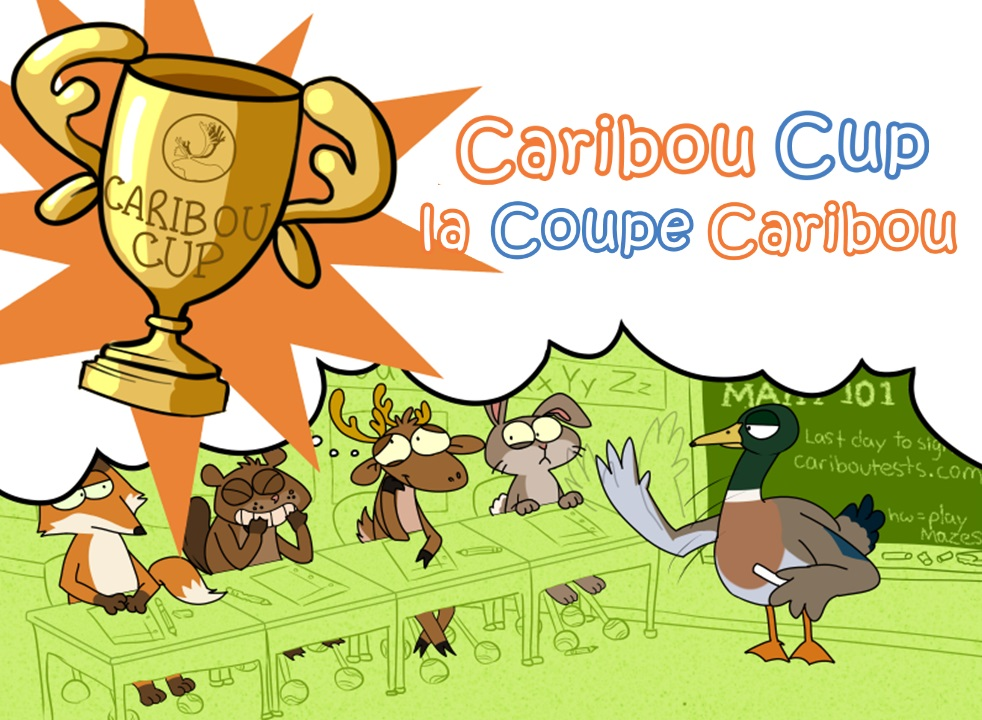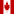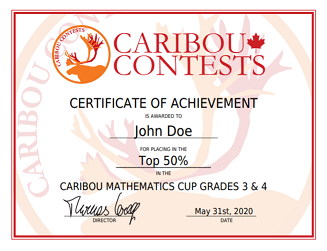Caribou in Covid: Contests are running online as usual. Check out the FAQ for further questions.

Prizes | Caribou Contests# Caribou Cup PrizesThe top performing students in the Caribou Cup are awarded cash prizes at the end of the six contests in the Cup (Oct - May). A student's Caribou Cup score is the sum of their best 5 out of the 6 contests. Prizes are awarded according to this sum. If two students have the same sum, then the total score in all 6 contests is used as the tie-breaker for the Cup ranking.

While most top-ranked students will receive a cash prize, if there are many students with the same top rank (particularly with lower grades) for the prize to be distributed fairly and feasibly, an algorithm randomly selects students from this rank to receive a prize. In other words, if there are 50 students in rank 3 with \$10 to split between them, we prefer to give \$5 to two randomly-selected students than to give \$0.20 to 50 students. For details, see the explanation below.

The amount of money given out as a prize is calculated as follows, where `score` is the percentage score the student got in the Caribou Cup (eg. 360 points out of a possible 480 = `0.75`).

Money amounts are in CAD.

RankStudent Prize
1st\$100 × `score`
2nd\$75 × `score`
3rd\$50 × `score`
4th and on\$30 × `score`

Prizes are awarded in this manner until the prize pool is empty. If for a given rank the total prize pool remaining for that rank is less than the total prize amount calculated based on the students' scores, then:

• if the total prize pool remaining ÷ number of students of that rank ≥ \$10, then the total prize pool remaining is split between all students of that rank
• if the total prize pool remaining ÷ number of students of that rank < \$10, then a number X of students, where X is the quotient of total prize pool remaining ÷ \$10 rounded down to the nearest integer, are chosen at random from that rank and given \$10

The prize pools for each grade level are as follows (subject to change). Note the K/1 level has no prize pool as participants are not ranked:

After the Caribou Cup Scores and Ranks are finalized, the algorithm determines the distribution of the Prize pools for each grade and we will contact the students' Caribou Coordinators via the emails they have provided about claiming the prizes. We offer both cheques and electronic gift cards, however the recipients must pay any cheque acceptance fees and conversion fees at their local bank, whereas electronic gift cards do not incur additional costs.

# Certificates

Each student is able to print a Certificate of Achievement and a Certificate of Participation for each Caribou Contest they participate in and for the Caribou Cup (after the last contest). To download/print certificates, the student or their Caribou Coordinator must log in and go to the Results section of their account. Click the buttons below to generate some sample Certificates of in English.# Participation Prizes

To reward effort and help encourage students to participate in Caribou Contests even if they are not necessarily in the top ranks, we have implemented a participation prize. This works like a draw where each contest written is one entry in the draw, and the selected students from each grade will receive a \$5 CAD gift card. The more contests you participate in, the higher your chances!